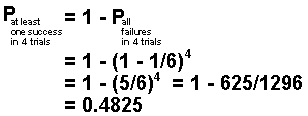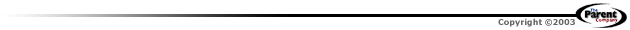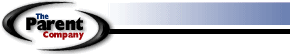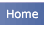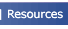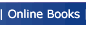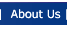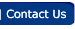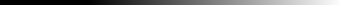Home \ Online Books \ The Creation Explanation The Creation Explanation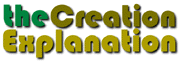Appendix D
 Some Basic Probability Theory If there are several different possible outcomes of any kind of operation, the sum of the probabilities of the various outcomes is equal to unity, i.e., the number 1. In this case, in N random constructions of a protein molecule the two possible outcomes are either (1) all failures to produce an active enzyme, i.e., zero successes, or (2) at least one success. Thus,Also note that if the probability of failure in one trial is Pf, the probability of failure for two trials is (Pf)2, and for failure all N times in N trials is (Pf)N. Thus, in rolling a six-sided die once, the probability of getting any particular result, say the number 3, 1/6. The probability of failing to get a 3 in one trial rolling of the die is 1-1/6 = 5/6. then in four trials, for example, the probability of never rolling a 3, i.e. of failing to get a 3 in at least one of the four trials is (1-1/6) = (5/6)4. So we conclude that in four trials the probability of succeeding in rolling a 3 at least once is